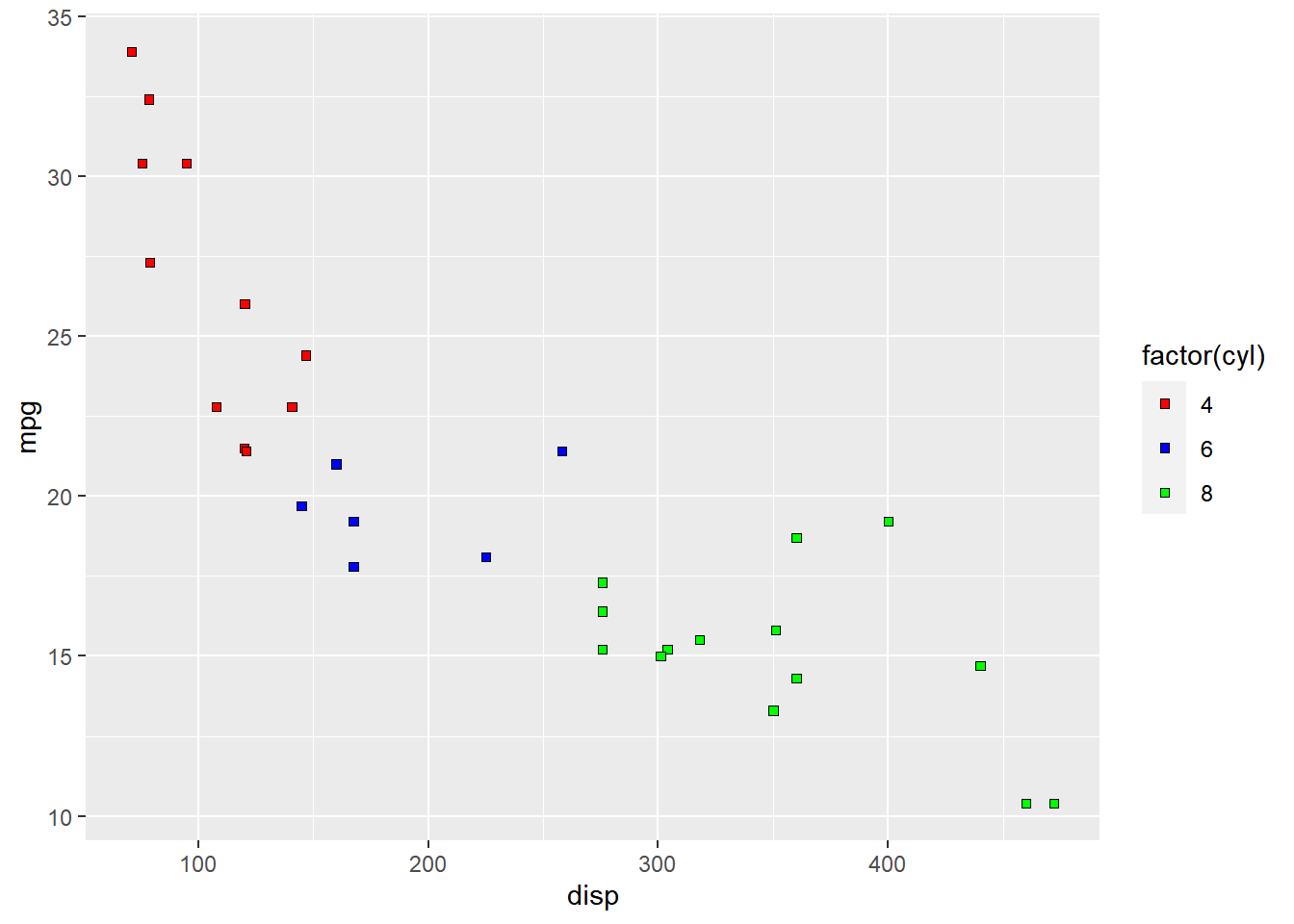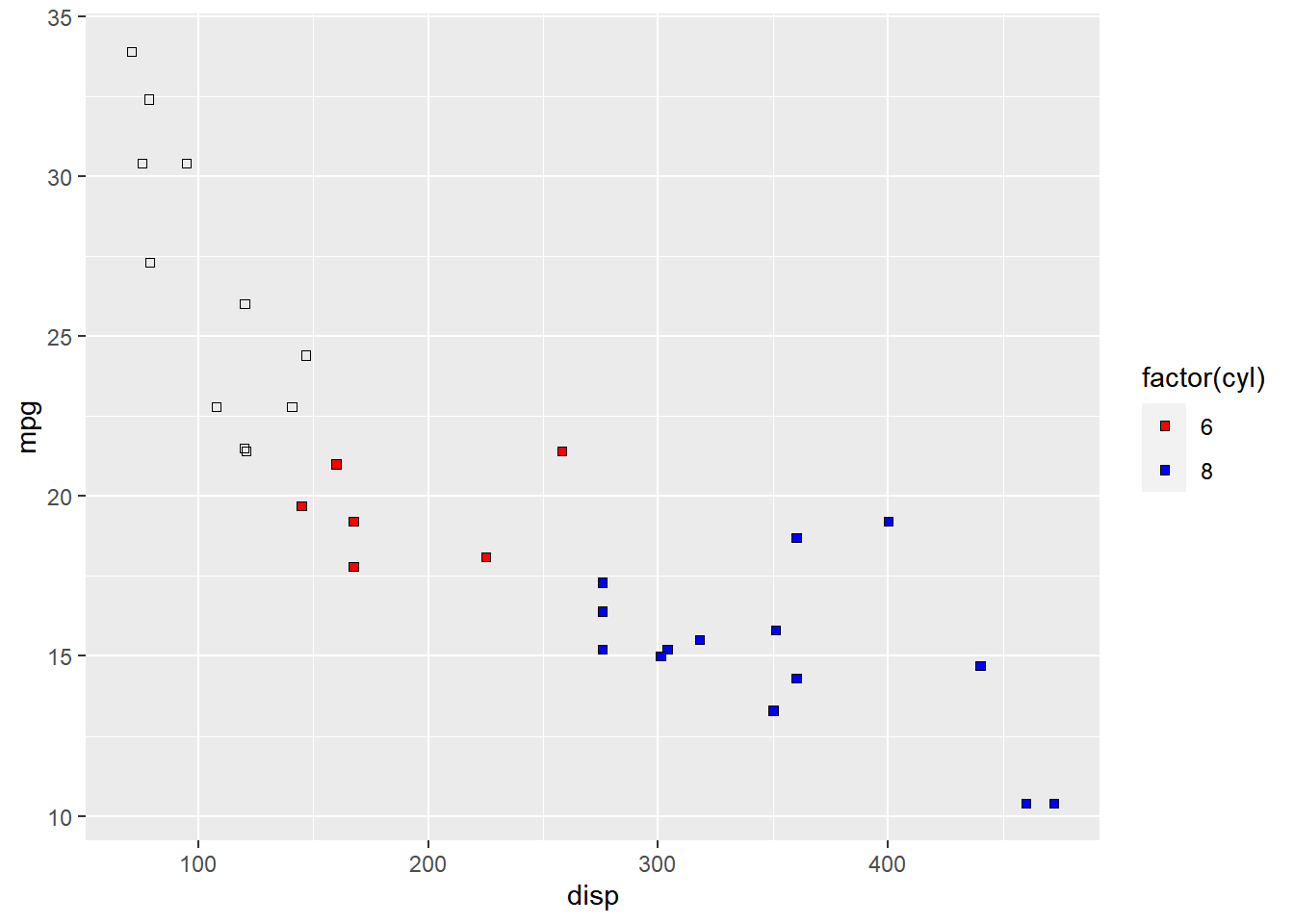Want to share your content on R-bloggers? click here if you have a blog, or here if you don't.

### Introduction

This is the 14th post in the series Elegant Data Visualization with ggplot2. In the previous post, we learnt how to modify the legend of plots when aesthetics are mapped to variables. In this post, we will continue to explore different ways to modify/customize the legend of plots.

Specifically, we will learn to modify the following using scale_fill_manual() when fill is mapped to categorical variables:

• title
• breaks
• limits
• labels
• values

### Libraries, Code & Data

We will use the following libraries in this post:

All the data sets used in this post can be found here and code can be downloaded from here.

### Plot

Let us start with a scatter plot examining the relationship between displacement and miles per gallon from the mtcars data set. We will map fill to the cyl variable.

ggplot(mtcars) +
geom_point(aes(disp, mpg, fill = factor(cyl)), shape = 22)As you can see, the legend acts as a guide for the color aesthetic. Now, let us learn to modify the different aspects of the legend.

### Title

The title of the legend (factor(cyl)) is not very intuitive. If the user does not know the underlying data, they will not be able to make any sense out of it. Let us change it to Cylinders using the name argument.

ggplot(mtcars) +
geom_point(aes(disp, mpg, fill = factor(cyl)), shape = 22) +
scale_fill_manual(name = "Cylinders",
values = c("red", "blue", "green"))### Values

To change the default colors in the legend, use the values argument and supply a character vector of color names. The number of colors specified must be equal to the number of levels in the categorical variable mapped. In the below example, cyl has 3 levels (4, 6, 8) and hence we have specified 3 colors.

ggplot(mtcars) +
geom_point(aes(disp, mpg, fill = factor(cyl)), shape = 22) +
scale_fill_manual(values = c("red", "blue", "green"))### Labels

The labels in the legend can be modified using the labels argument. Let us change the labels to Four, Six and Eight in the next example. Ensure that the labels are intuitive and easy to interpret for the end user of the plot.

ggplot(mtcars) +
geom_point(aes(disp, mpg, fill = factor(cyl)), shape = 22) +
scale_fill_manual(values = c("red", "blue", "green"),
labels = c('Four', 'Six', 'Eight'))### Limits

Let us assume that we want to modify the data to be displayed i.e. instead of examining the relationship between mileage and displacement for all cars, we desire to look at only cars with at least 6 cylinders. One way to approach this would be to filter the data using filter from dplyr and then visualize it. Instead, we will use the limits argument and filter the data for visualization.

ggplot(mtcars) +
geom_point(aes(disp, mpg, fill = factor(cyl)), shape = 22) +
scale_fill_manual(values = c("red", "blue", "green"),
limits = c(6, 8))As you can see above, ggplot2 returns a warning message indicating data related to 4 cylinders has been dropped. If you observe the legend, it now represents only 4 and 6 cylinders.

### Breaks

When there are large number of levels in the mapped variable, you may not want the labels in the legend to represent all of them. In such cases, we can use the breaks argument and specify the labels to be used. In the below case, we use the breaks argument to ensure that the labels in legend represent two levels (4, 8) of the mapped variable.

ggplot(mtcars) +
geom_point(aes(disp, mpg, fill = factor(cyl)), shape = 22) +
scale_fill_manual(values = c("red", "blue", "green"),
breaks = c(4, 8))### Putting it all together…

ggplot(mtcars) +
geom_point(aes(disp, mpg, fill = factor(cyl)), shape = 22) +
scale_fill_manual(name = "Cylinders", values = c("red", "blue", "green"),
labels = c('Four', 'Six', 'Eight'), limits = c(4, 6, 8), breaks = c(4, 6, 8))### Summary

In this post, we learnt to modify the following aspects of legends:

• title
• breaks
• limits
• labels
• values

### Up Next..

In the next post, we will learn to modify the legend when shape is mapped to categorical variables.